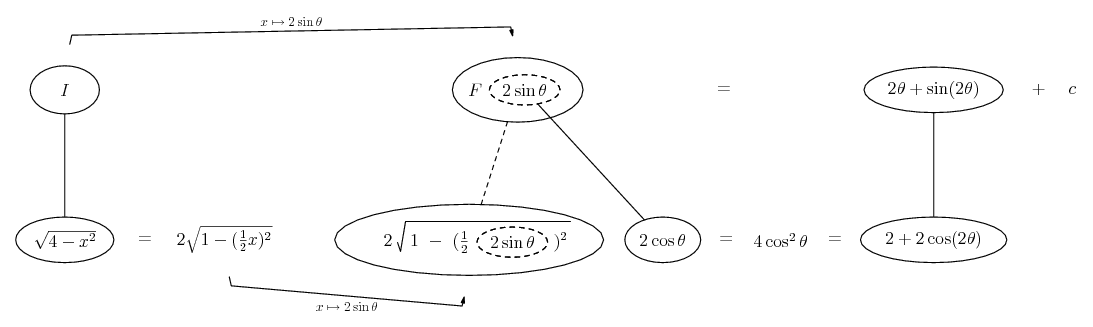# Thread: Trigonometric and Hyperbolic substitutions

1. ## Trigonometric and Hyperbolic substitutions

So confused at this. I was sick when the class went through it and now I'm just looking at the questions with a blank face.Right, so here's what they give us:

If for some constant a the integrand contains:
$\displaystyle a^{2} - x^{2}$, let $\displaystyle x = a \sin{\theta}$ with $\displaystyle dx = a \cos{\theta}d\theta$

$\displaystyle a^{2} + x^{2}$, let $\displaystyle x = a \tan{\theta}$ with $\displaystyle dx = a \sec^2{\theta}d\theta$

$\displaystyle x^{2} - a^{2}$, let $\displaystyle x = a \sec{\theta}$ with $\displaystyle dx = a \sec{\theta}\tan{\theta}d\theta$

$\displaystyle \sqrt{x^{2} + a^{2}}$, let $\displaystyle x = a \sinh{t}$ with $\displaystyle dx = a \cosh{t}dt$

$\displaystyle \sqrt{x^{2} - a^{2}}$, let $\displaystyle x = a \cosh{t}$ with $\displaystyle dx = a \sinh{t}dt$

They have a sample solution that I can't even follow.$\displaystyle \int{\sqrt{4 - x^{2}},dx}$
I get that 4 is 2^2, so 2 is a.

= $\displaystyle \int{\sqrt{4(1 - \sin^2{\theta})}2\cos{\theta}\,d\theta}$
Starting to lose track here. AFAIK, x should be 2 sin theta, though that doesn't explain why the 4 has been taken out.

= $\displaystyle \int{4\sqrt{\cos^2{\theta}}\cos{\theta}\,d\theta}$
Confused by this stage. =|

= $\displaystyle \int{4\cos^2{\theta},d\theta}$
Confused.

= $\displaystyle \int{2(1 + cos{\theta},d\theta}$
I'm pretty sure that's 2 times 2cos^2(theta), so I 'kinda' get this step. Still not sure how it got to this stage.

= $\displaystyle 2\theta + \sin{\theta} + C$

Because $\displaystyle x = 2\sin{\theta}$, $\displaystyle \theta = \arcsin{\frac{x}{2}}$

$\displaystyle \sin{2\theta} = 2 \sin{\theta}\cos{\theta}$
= $\displaystyle 2 \sin{\theta} \sqrt{1 - \sin^2{\theta}}$
= $\displaystyle x\sqrt{1 - (\frac{x}{2})^2}$

Therefore $\displaystyle \int{\sqrt{4 - x^{2}},dx} = 2 \arcsin{\frac{x}{2}} + x\sqrt{1 - (\frac{x}{2})^2}$

So yes, I essentially have no clue about how they got there.Anybody feel up to trying to explain this to me?

2. Just in case a picture helps...... where... is the chain rule. Straight continuous lines differentiate downwards (integrate up) with respect to x, and the straight dashed line similarly but with respect to the dashed balloon expression (the inner function of the composite which is subject to the chain rule).

Hope that might help - follow round clockwise from bottom left. Full size pic in here:

Spoiler:By the way, you're aware of Pythagoras' involvement for simplifying to $\displaystyle 4 \cos^2 \theta$ ?

__________________________________________

Don't integrate - balloontegrate!

Balloon Calculus: Gallery

Balloon Calculus Drawing with LaTeX and Asymptote!

3. I'm going to fill in the intermediate steps for you

we have $\displaystyle \int{\sqrt{4 - x^{2}} dx}$ and $\displaystyle x = 2\sin{\theta} \rightarrow dx = 2\cos{\theta}d\theta$

so we get by substituting x and dx into the integral
$\displaystyle \int{\sqrt{4 - (2\sin{\theta})^{2}}*2\cos{\theta}d\theta} = \int{\sqrt{4 - 4\sin^2{\theta}}*2\cos{\theta}d\theta} $$\displaystyle = \int{\sqrt{4(1 - \sin^2{\theta})}*2\cos{\theta}d\theta} = \int{2\sqrt{(1 - \sin^2{\theta})}*2\cos{\theta}d\theta}$$\displaystyle = \int{4\sqrt{(1 - \sin^2{\theta})}*\cos{\theta}d\theta}$

since $\displaystyle \sin^2{\theta} + \cos^2{\theta} = 1 \rightarrow 1 - \sin^2{\theta} = \cos^2{\theta}$
so, $\displaystyle \int{4\sqrt{(1 - \sin^2{\theta})}*\cos{\theta}d\theta} = \int{4\sqrt{\cos^2{\theta}}*\cos{\theta}d\theta} = \int{4\cos{\theta}*\cos{\theta}d\theta}$$\displaystyle = \int{4\cos^2{\theta}d\theta} Now we use the fact that \displaystyle \cos^2{\theta} = \frac{1}{2}(1 + \cos{2\theta}) \displaystyle \int{4\cos^2{\theta}d\theta} = \int{4(\frac{1}{2}(1 + \cos{2\theta}))d\theta}$$\displaystyle = \int{2(1 + \cos{2\theta})d\theta}= \int{2 + 2\cos{2\theta}d\theta}$$\displaystyle = 2\theta + \sin{2\theta} + C$

Now we undo our substitution. since $\displaystyle x = 2\sin{\theta} \rightarrow \theta = \arcsin{\frac{x}{2}}$

We use the fact that $\displaystyle \sin{2\theta} = (2\sin{\theta})\cos{\theta}$
and we get $\displaystyle (2\sin{\theta})\cos{\theta} = (2\sin{\theta})\sqrt{\cos^2{\theta}} = (2\sin{\theta})\sqrt{1 - \sin^2{\theta}} = x*\sqrt{1 - {(\frac{x}{2}})^2}$

so we have
$\displaystyle \int{\sqrt{4 - x^{2}} dx} = 2\theta + \sin{2\theta} + C = \arcsin{\frac{x}{2}} + x*\sqrt{1 - {(\frac{x}{2}})^2} + C$

#### Search Tags

hyperbolic, substitutions, trigonometric